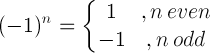# Exponent Rules

The base a is raised to the power of n is equal to the multiplication of a, n times:

an = a × a ×× a

n times

a is the base and n is the exponent.

Exponents rules and properties

Rule name
Rule
Example
Product rules
a n · a m = a n+m
23 · 24 = 23+4 = 128

a n · b n = (a · b) n
32 · 42 = (3·4)2 = 144
Quotient rules
a n / a m = a nm
25 / 23 = 25-3 = 4

a n / b n = (a / b) n
43 / 23 = (4/2)3 = 8
Power rules
(bn)m = bn·m
(23)2 = 23·2 = 64

bnm = b(nm)
232 = 2(32)= 512

m√(bn) = b n/m
2√(26) = 26/2 = 8

b1/n = nb
81/3 = 3√8 = 2
Negative exponents
b-n = 1 / bn
2-3 = 1/23 = 0.125
Zero rules
b0 = 1
50 = 1

0n = 0 , for n>0
05 = 0
One rules
b1 = b
51 = 5

1n = 1
15 = 1
Minus one rule(-1)5 = -1
Derivative rule
(xn)= n·x n-1
(x3)= 3·x3-1
Integral rule
xndx = xn+1/(n+1)+C
x2dx = x2+1/(2+1)+C

### Exponents product rules

#### Product rule with same base

an · am = an+m

Example:

23 · 24 = 23+4 = 27 = 2·2·2·2·2·2·2 = 128

#### Product rule with same exponent

an · bn = (a · b)n

Example:

32 · 42 = (3·4)2 = 122 = 12·12 = 144

### Exponents quotient rules

#### Quotient rule with same base

an / am = anm

Example:

25 / 23 = 25-3 = 22 = 2·2 = 4

#### Quotient rule with same exponent

an / bn = (a / b)n

Example:

43 / 23 = (4/2)3 = 23 = 2·2·2 = 8

### Exponents power rules

#### Power rule I

(an) m = a n·m

Example:

(23)2 = 23·2 = 26 = 2·2·2·2·2·2 = 64

#### Power rule II

anm = a(nm)

Example:

232 = 2(32) = 2(3·3) = 29 = 2·2·2·2·2·2·2·2·2 = 512

#### Power rule with radicals

m√(a n) = a n/m

Example:

2√(26) = 26/2 = 23 = 2·2·2 = 8

### Negative exponents rule

b-n = 1 / bn

Example:

2-3 = 1/23 = 1/(2·2·2) = 1/8 = 0.125